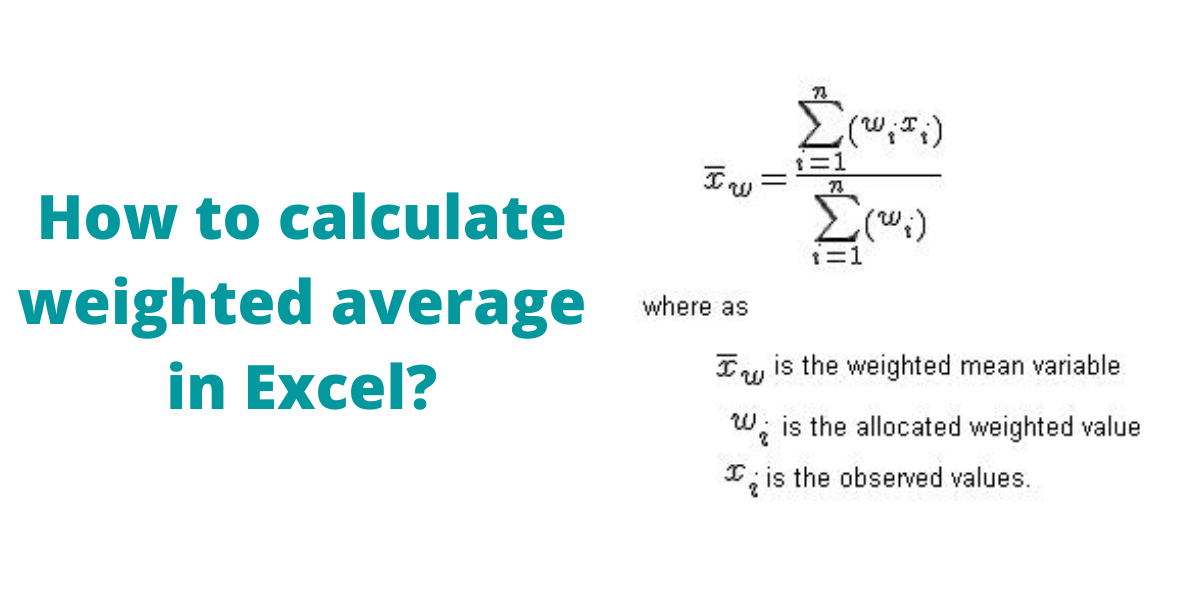# How to Calculate the Weighted Average in Excel?A weighted average is an arithmetic mean in which certain data elements in a dataset are more significant than others. A greater significance or weight is assigned to one or more numbers. Although there is no built-in method to calculate weighted average in Microsoft Excel, functions like SUMPRODUCT and SUM can be utilized to do the same.

Recommended read: Calculate the Square Root in Excel

## What is Weighted Average?

Let us take an example, where a student gets marks in different evaluation components and needs to be given a final score or grade. A simple average can not be calculated here as the weightage of different evaluation components varies.

Here, to find the weighted average, we need to multiply marks with their corresponding weightages, add them together and then divide by the sum total of weightages.

## Quick Overview of the SUMPRODUCT and SUM function

The function SUMPRODUCT returns the sum of the products of the corresponding arrays or ranges.

Syntax: `=SUMPRODUCT(array1, [array2], [array3], ...)`, where array1, array2,… arrayN are the arrays whose corresponding components you want to first multiply and then add.

The SUM function simply adds values or values in the range given as arguments.

Syntax: `=SUM(n)`, where n is the list of numbers or cell ranges in the form of A1:A10.

## Calculate the Weighted Average Using Excel

• Select the cell where you want to display the weighted average result.
• Type the formula:` =SUMPRODUCT(B2:B8,C2:C8)/SUM(C2:C8)`, where B2:B8 is the range of cells containing marks obtained and C2:C8 is the range of cells containing weights. Press Enter key to display the result.

## Conclusion

In this tutorial, we learned how to easily calculate weighted average in Excel using two inbuilt functions SUMPRODUCT and SUM.